# 机器学习|梯度下降详解

### 线性回归

$$\hat{y} = \theta_0 + \theta_1x_{1} + \theta_2x_{2} + … + \theta_nx_{n}$$

• $\hat{y}$ 是预测值
• $n$ 是特征的数量
• $x_i$ 是 $i$ 个特征值
• $θ_j$ 是第 $j$ 个模型参数 (包括偏置项 $θ_0$ 以及特征权重 $θ_1,θ_2,…,θ_n$

$$\hat{y} = h_{\theta}(X) = \theta^T \cdot X$$

• $θ$ 是模型的参数向量，包括偏置项 $θ_0$ 以及特征权重 $θ_1$ 到 $θ_n$
• $θ^T$ 是 $θ$ 的转置向量 (为行向量，而不再是列向量)
• $X$ 是实例的特征向量，包括从 $θ_0$ 到 $θ_n,θ_0$ 永远为 $1$
• $θ^T⋅X$ 是 $θ^T$ 和 $X$ 的点积
• $h_θ$ 是模型参数 $θ$ 的假设函数

$$MSE(X, h_{\theta}) = \frac{1}{m}\sum_{i=1}^{m} (\theta^T \cdot X^{(i)} - y^{(i)})^2$$

### 标准方程

$$MSE(X, h_{\theta}) = \frac{1}{m}\sum_{i=1}^{m} (\theta^T \cdot X^{(i)} - y^{(i)})^2$$

• $\hat{\theta}$ 是使成本函数最小的 $θ$ 值
• $y$ 是包含 $y^{(1)}$ 到 $y^{(m)}$ 的目标值量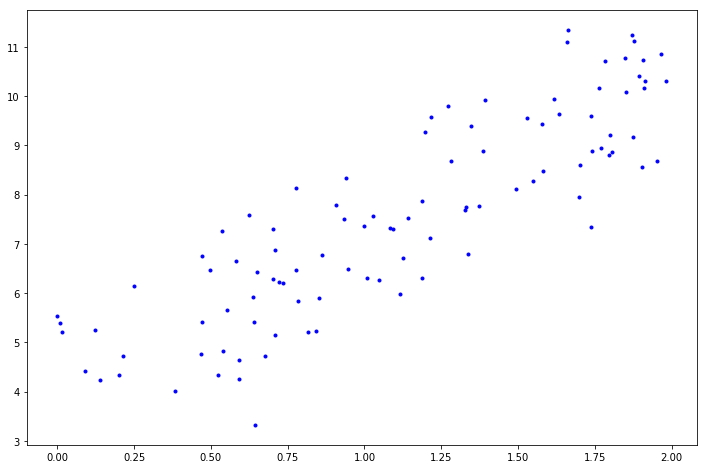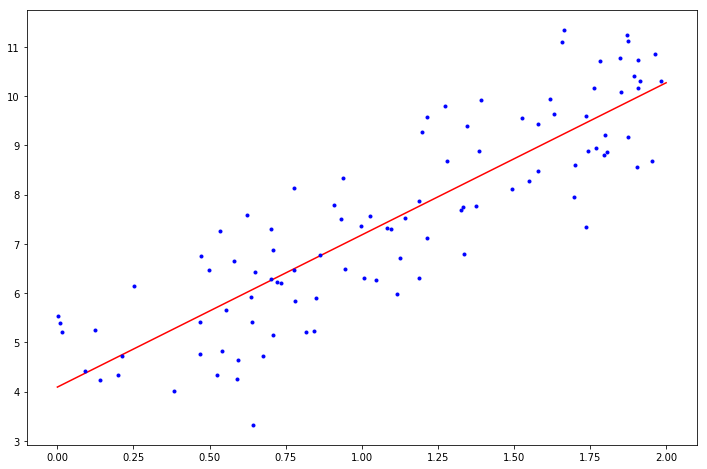### 梯度下降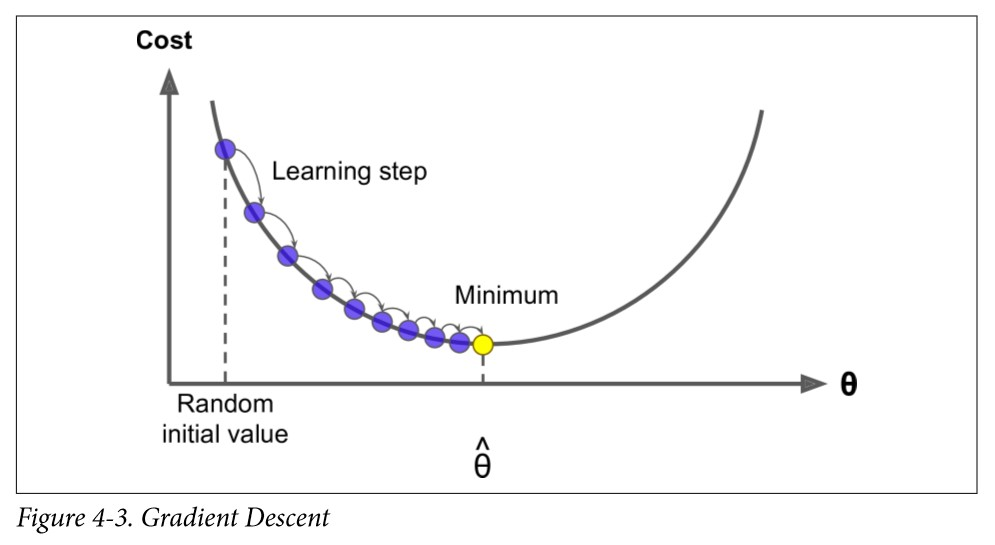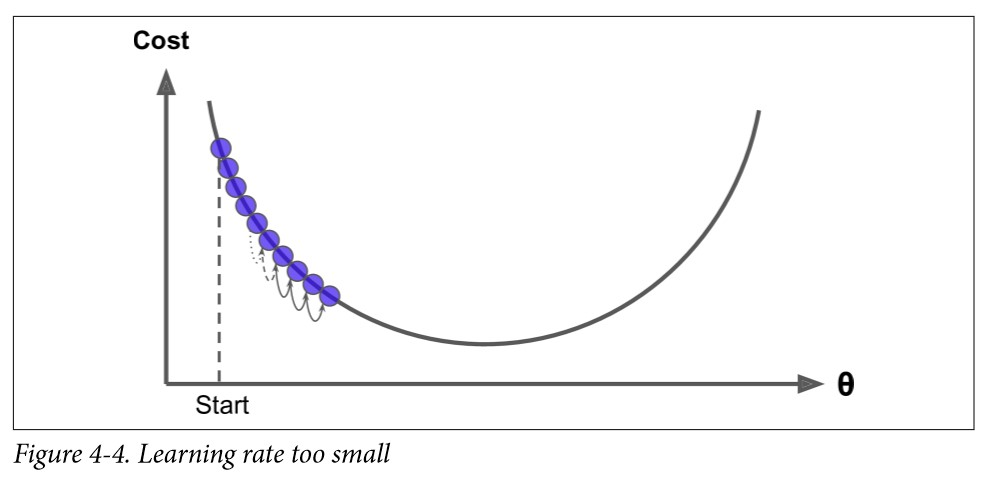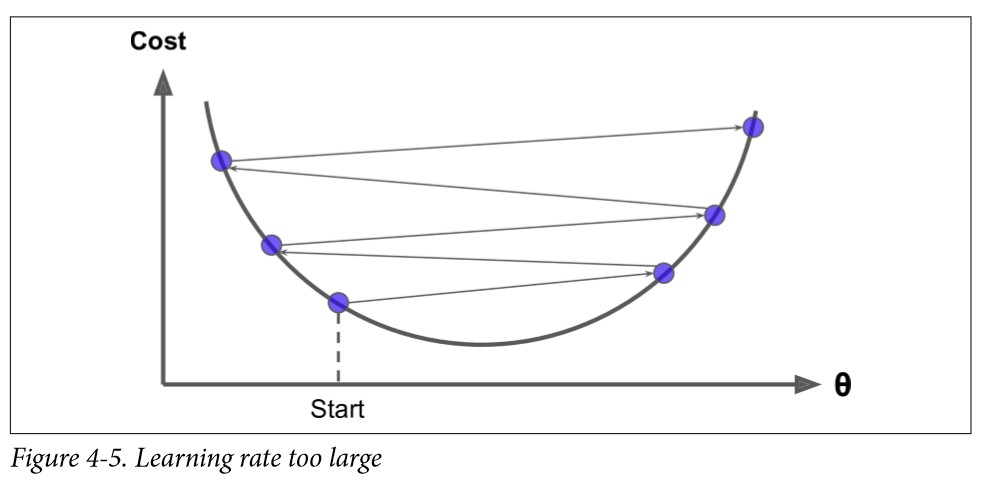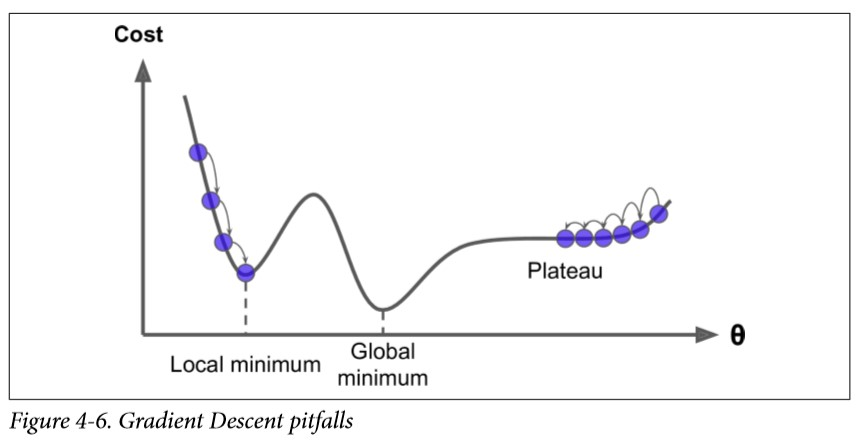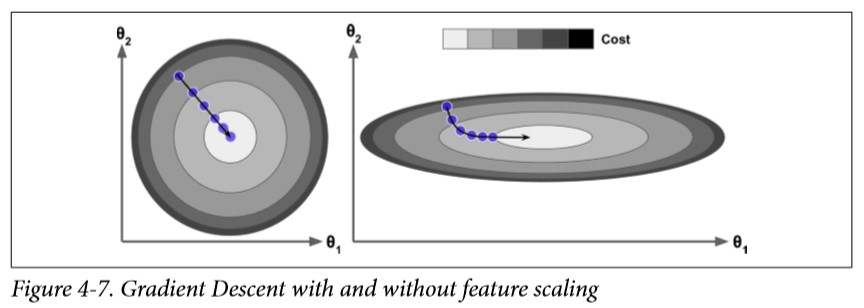### 批量梯度下降

$$\frac{\partial}{\partial \theta_j}MSE(\theta) = \frac{2}{m}\sum_{i=1}^{m} (\theta^T \cdot X^{(i)} - y^{(i)})x_j^{(i)}$$

$$\nabla_\theta MSE(\theta) = \left[ \begin{matrix} \frac{\partial}{\partial \theta_0}MSE(\theta) \ \frac{\partial}{\partial \theta_1}MSE(\theta) \ … \ \frac{\partial}{\partial \theta_n}MSE(\theta) \end{matrix} \right] = \frac{2}{m}X^T \cdot (X \cdot \theta - y)$$

$$\theta^{(next step)} = \theta - \eta\nabla_\theta MSE(\theta)$$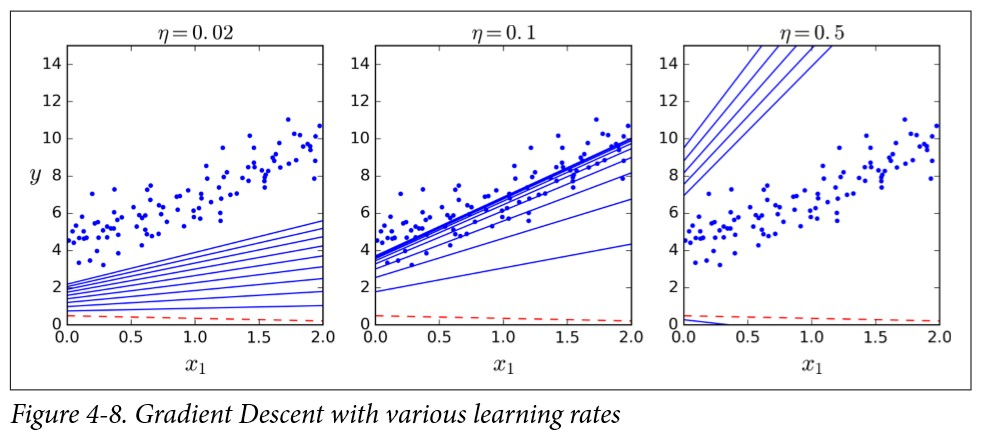### 随机梯度下降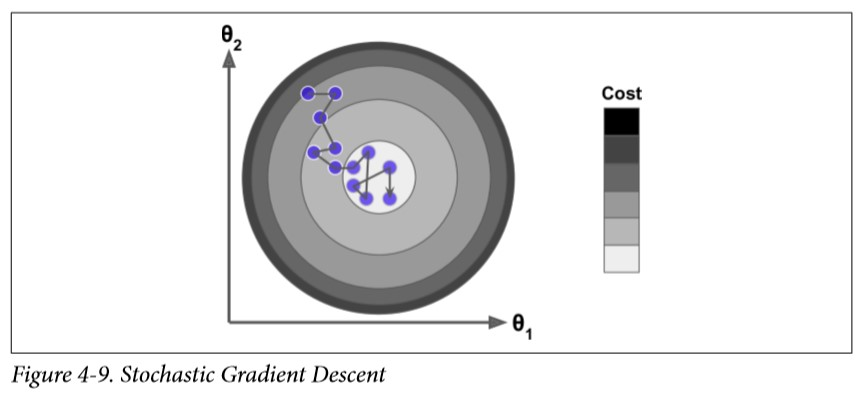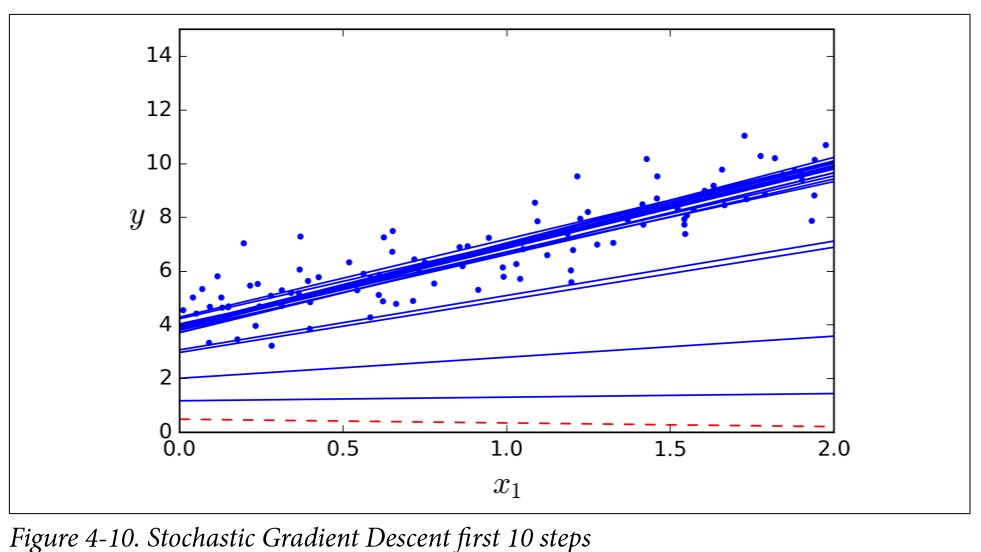Scikit-Learn 里，用 $SGD$ 执行线性回归可以使用 SGDRegressor 类，其默认优化的成本函数是平方误差。下面这段代码从学习率 0.1 开始（eta0=0.1），使用默认的学习计划（跟前面的学习计划不同） 运行了50 轮，而且没有使用任何正则化（penalty=None）：

### 小批量梯度下降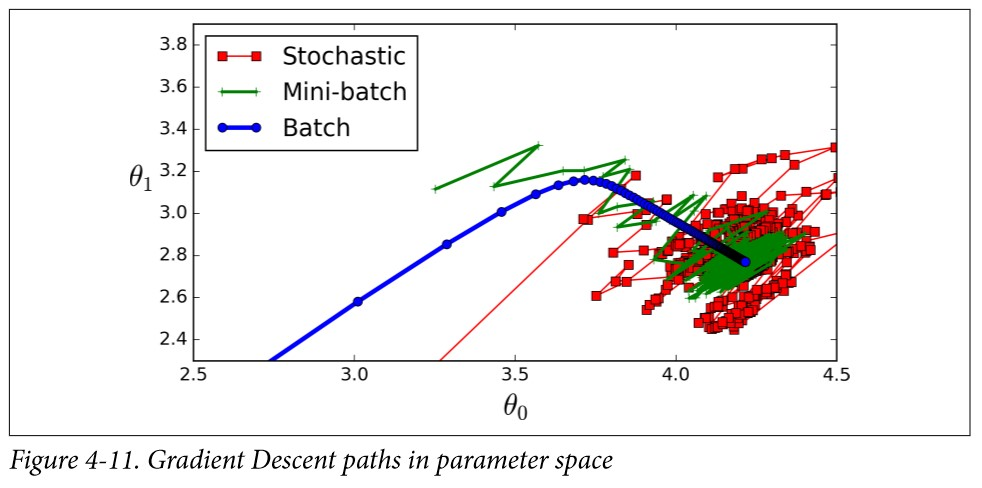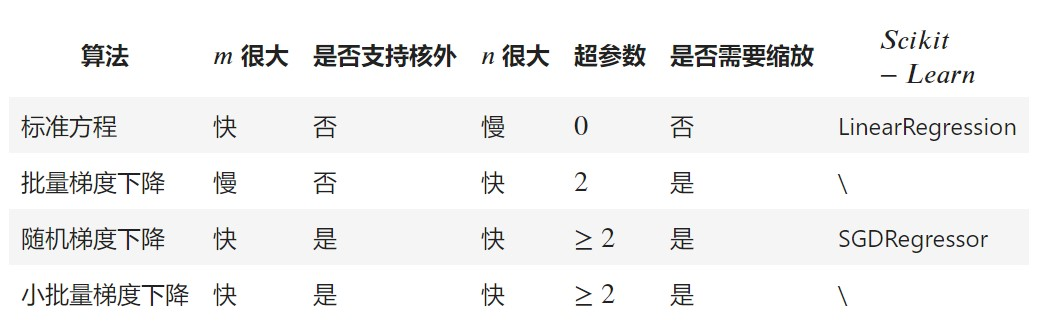_参考_：

laugh12321

2019-03-12

2020-10-24